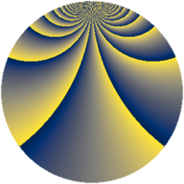# Properties

 Label 2880.2.brLevel $2880$ Weight $2$ Character orbit 2880.br Rep. character $\chi_{2880}(959,\cdot)$ Character field $\Q(\zeta_{6})$ Dimension $280$ Sturm bound $1152$

# Learn more about

## Defining parameters

 Level: $$N$$ $$=$$ $$2880 = 2^{6} \cdot 3^{2} \cdot 5$$ Weight: $$k$$ $$=$$ $$2$$ Character orbit: $$[\chi]$$ $$=$$ 2880.br (of order $$6$$ and degree $$2$$) Character conductor: $$\operatorname{cond}(\chi)$$ $$=$$ $$180$$ Character field: $$\Q(\zeta_{6})$$ Sturm bound: $$1152$$

## Dimensions

The following table gives the dimensions of various subspaces of $$M_{2}(2880, [\chi])$$.

Total New Old
Modular forms 1200 296 904
Cusp forms 1104 280 824
Eisenstein series 96 16 80

## Trace form

 $$280q + 6q^{5} - 8q^{9} + O(q^{10})$$ $$280q + 6q^{5} - 8q^{9} - 4q^{21} - 2q^{25} + 12q^{29} - 12q^{41} - 10q^{45} - 120q^{49} + 4q^{61} - 6q^{65} - 28q^{69} + 8q^{81} + 12q^{85} + O(q^{100})$$

## Decomposition of $$S_{2}^{\mathrm{new}}(2880, [\chi])$$ into newform subspaces

The newforms in this space have not yet been added to the LMFDB.

## Decomposition of $$S_{2}^{\mathrm{old}}(2880, [\chi])$$ into lower level spaces

$$S_{2}^{\mathrm{old}}(2880, [\chi]) \cong$$ $$S_{2}^{\mathrm{new}}(180, [\chi])$$$$^{\oplus 5}$$$$\oplus$$$$S_{2}^{\mathrm{new}}(720, [\chi])$$$$^{\oplus 3}$$$$\oplus$$$$S_{2}^{\mathrm{new}}(1440, [\chi])$$$$^{\oplus 2}$$